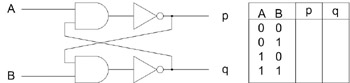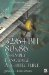Exercises1. What is a half- adder ?

2. Make a NOT gate using

1. an XOR gate

2. a NAND gate

3. a NOR gate.

3. Write a software algorithm for the following equation. D=(1(A B)) (A B)

What does the equation do?

4. Draw an XOR circuit using only OR gates and AND gates, and a subtraction.

5. Make a 128-bit XOR using only bitwise OR and bitwise AND, and a subtraction.

6. Write code to change the value of tri-state data. Note the series of states is a repeating pattern {0, 1, 2, 0, 1, 2, }. The starting state is State #0.

State

1

2

Value

0x34

0x8A

0xE5

0x34

Extra credit:This cross- coupled SR flip-flop will be more familiar to those of you with electronics experience. Using A=0 and B=0 as the starting state, finish the logic table.

How does this circuit function? What are the behaviors of p and q if the data inputs of A and B are changed to a new state? Try all 12 possibilities. How would you code it?32/64-Bit 80x86 Assembly Language Architecture
ISBN: 1598220020
EAN: 2147483647
Year: 2003
Pages: 191

Similar book on Amazon